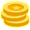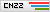# 【技术分享】Lua程序逆向之Luac字节码与反汇编

|评论1

|Luac指令在Lua中使用Instruction来表示，是一个32位大小的数值。在Luac.bt中，我们将其定义了为Inst结构体，回顾一下它的定义与读取函数：

``````typedef struct(int pc) {
local int pc_ = pc;
local uchar inst_sz = get_inst_sz();
if (inst_sz == 4) {
uint32 inst;
} else {
Warning("Error size_Instruction.");
}
} Inst <optimize=false>;``````

Luac指令完整由：OpCode、OpMode操作模式，以及不同模式下使用的不同的操作数组成。

``enum OpMode {iABC, iABx, iAsBx, iAx};``

ABC这些字节大小与起始位置的定义如下：

``````#define SIZE_C9
#define SIZE_B9
#define SIZE_Bx(SIZE_C + SIZE_B)
#define SIZE_A8
#define SIZE_Ax(SIZE_C + SIZE_B + SIZE_A)
#define SIZE_OP6
#define POS_OP0
#define POS_A(POS_OP + SIZE_OP)
#define POS_C(POS_A + SIZE_A)
#define POS_B(POS_C + SIZE_C)
#define POS_BxPOS_C
#define POS_AxPOS_A``````

``````typedef enum {
/*----------------------------------------------------------------------
nameargsdescription
------------------------------------------------------------------------*/
OP_MOVE,/*A BR(A) := R(B)*/
OP_LOADBOOL,/*A B CR(A) := (Bool)B; if (C) pc++*/
OP_LOADNIL,/*A BR(A) := ... := R(B) := nil*/
OP_GETUPVAL,/*A BR(A) := UpValue[B]*/
OP_GETGLOBAL,/*A BxR(A) := Gbl[Kst(Bx)]*/
OP_GETTABLE,/*A B CR(A) := R(B)[RK(C)]*/
OP_SETGLOBAL,/*A BxGbl[Kst(Bx)] := R(A)*/
OP_SETUPVAL,/*A BUpValue[B] := R(A)*/
OP_SETTABLE,/*A B CR(A)[RK(B)] := RK(C)*/
......
OP_CLOSE,/*A close all variables in the stack up to (>=) R(A)*/
OP_CLOSURE,/*A BxR(A) := closure(KPROTO[Bx], R(A), ... ,R(A+n))*/
OP_VARARG/*A BR(A), R(A+1), ..., R(A+B-1) = vararg*/
} OpCode;``````

OpCode定义的注释中，详细说明了每一条指令的格式、使用的参数，以及它的含义。以第一条OP_MOVE指令为例，它接受两个参数R(A)与R(B)，的作用是完成一个赋值操作“R(A) := R(B)”

``````LUAI_DDEF const lu_byte luaP_opmodes[NUM_OPCODES] = {
/*       T  A    B       C     mode   opcode*/
opmode(0, 1, OpArgR, OpArgN, iABC)/* OP_MOVE */
,opmode(0, 1, OpArgK, OpArgN, iABx)/* OP_LOADK */
,opmode(0, 1, OpArgN, OpArgN, iABx)/* OP_LOADKX */
,opmode(0, 1, OpArgU, OpArgU, iABC)/* OP_LOADBOOL */
,opmode(0, 1, OpArgU, OpArgN, iABC)/* OP_LOADNIL */
,opmode(0, 1, OpArgU, OpArgN, iABC)/* OP_GETUPVAL */
,opmode(0, 1, OpArgU, OpArgK, iABC)/* OP_GETTABUP */
,opmode(0, 1, OpArgR, OpArgK, iABC)/* OP_GETTABLE */
,opmode(0, 0, OpArgK, OpArgK, iABC)/* OP_SETTABUP */
,opmode(0, 0, OpArgU, OpArgN, iABC)/* OP_SETUPVAL */
,opmode(0, 0, OpArgK, OpArgK, iABC)/* OP_SETTABLE */
,opmode(0, 1, OpArgU, OpArgU, iABC)/* OP_NEWTABLE */
,opmode(0, 1, OpArgR, OpArgK, iABC)/* OP_SELF */
,opmode(0, 1, OpArgK, OpArgK, iABC)/* OP_ADD */
,opmode(0, 1, OpArgK, OpArgK, iABC)/* OP_SUB */
......
,opmode(0, 1, OpArgU, OpArgN, iABx)/* OP_CLOSURE */
,opmode(0, 1, OpArgU, OpArgN, iABC)/* OP_VARARG */
,opmode(0, 0, OpArgU, OpArgU, iAx)/* OP_EXTRAARG */
};``````

``#define opmode(t,a,b,c,m) (((t)<<7) | ((a)<<6) | ((b)<<4) | ((c)<<2) | (m))``

m位，占最低2位，即前面OpMode中定义的四种模式，通过它，可以确定OpCode的参数部分。

``````enum OpArgMask {
OpArgN,  /* 参数未被使用 */
OpArgU,  /* 已使用参数 */
OpArgR,  /* 参数是寄存器或跳转偏移 */
OpArgK   /* 参数是常量或寄存器常量 */
};``````

a位，占位6。表示是否是寄存器操作。

t位，占位7。表示是否是测试操作。跳转和测试指令该位为1。

luaP_opmodes的值使用如下代码打印出来：

``````printf("opcode ver 5.2:n");
for (int i=0; i<sizeof(luaP_opmodes); i++) {
printf("0x%x, ", luaP_opmodes[i]);
}
printf("n");``````

``````opcode ver 5.2:
0x60, 0x71, 0x41, 0x54, 0x50, 0x50, 0x5c, 0x6c, 0x3c, 0x10, 0x3c, 0x54, 0x6c, 0x7c, 0x7c, 0x7c, 0x7c, 0x7c, 0x7c, 0x60, 0x60, 0x60, 0x68, 0x22, 0xbc, 0xbc, 0xbc, 0x84, 0xe4, 0x54, 0x54, 0x10, 0x62, 0x62, 0x4, 0x62, 0x14, 0x51, 0x50, 0x17,``````

OpCode获取

``````#define GET_OPCODE(i)

``````uchar GET_OPCODE(uint32 inst) {
return ((inst)>>POS_OP) & ((~((~(Instruction)0)<<(SIZE_OP)))<<(0));
}``````

``````#define GETARG_A(i)getarg(i, POS_A, SIZE_A)
#define GETARG_B(i)getarg(i, POS_B, SIZE_B)
#define GETARG_C(i)getarg(i, POS_C, SIZE_C)
#define GETARG_Bx(i)getarg(i, POS_Bx, SIZE_Bx)
#define GETARG_Ax(i)getarg(i, POS_Ax, SIZE_Ax)
#define GETARG_sBx(i)(GETARG_Bx(i)-MAXARG_sBx)``````

``````int GETARG_A(uint32 inst) {
return ((inst)>>POS_A) & ((~((~(Instruction)0)<<(SIZE_A)))<<(0));
}
int GETARG_B(uint32 inst) {
return ((inst)>>POS_B) & ((~((~(Instruction)0)<<(SIZE_B)))<<(0));
}
int GETARG_C(uint32 inst) {
return ((inst)>>POS_C) & ((~((~(Instruction)0)<<(SIZE_C)))<<(0));
}
int GETARG_Bx(uint32 inst) {
return ((inst)>>POS_Bx) & ((~((~(Instruction)0)<<(SIZE_Bx)))<<(0));
}
int GETARG_Ax(uint32 inst) {
return ((inst)>>POS_Ax) & ((~((~(Instruction)0)<<(SIZE_Ax)))<<(0));
}
int GETARG_sBx(uint32 inst) {
return GETARG_Bx(inst)-MAXARG_sBx;
}``````

``````// luadec_disassemble() from luadec disassemble.c
local int i = inst.inst;
OpCode o = (OpCode)GET_OPCODE(i);
/*
Printf("inst: 0x%xn", o);
*/
local int a = GETARG_A(i);
local int b = GETARG_B(i);
local int c = GETARG_C(i);
local int bc = GETARG_Bx(i);
local int sbc = GETARG_sBx(i);
local int dest;
local string line;
local string lend;
local string tmpconstant1;
local string tmpconstant2;
local string tmp;
local string tmp2;
local uchar lua_version_num = get_lua_version();
local int pc = inst.pc_;

//Printf("Inst: %sn", EnumToString(o));
switch (o) {
case OP_MOVE:
/*	A B	R(A) := R(B)					*/
SPrintf(line,"R%d R%d",a,b);
SPrintf(lend,"R%d := R%d",a,b);
break;
/*	A Bx	R(A) := Kst(Bx)					*/
SPrintf(line,"R%d K%d",a,bc);
tmpconstant1 = DecompileConstant(parentof(parentof(inst)),bc);
SPrintf(lend,"R%d := %s",a,tmpconstant1);
break;
......
case OP_CLOSURE:
{
/*	A Bx	R(A) := closure(KPROTO[Bx])		*/
SPrintf(line,"R%d %d",a,bc);
SPrintf(lend, "R%d := closure(Function #%d)", a, bc);
break;
}
default:
break;

}

local string ss;
SPrintf(ss, "[%d] %-9s %-13s; %sn", pc, get_opcode_str(o),line,lend);

return ss;
}``````

``DecompileConstant(parentof(parentof(inst))``

``````typedef struct(string level) {
local string level_ = level;
//Printf("level:%sn", level_);
//code
//Code code;
struct Code {
uint32 sizecode <format=hex>;
local uchar inst_sz = get_inst_sz();
local int pc = 1;
if (inst_sz == 4) {
local uint32 sz = sizecode;
while (sz-- > 0) {
Inst inst(pc);
pc++;
}
} else {
Warning("Error size_Instruction.");
}

typedef struct(int pc) {
local int pc_ = pc;
local uchar inst_sz = get_inst_sz();
if (inst_sz == 4) {
uint32 inst;
} else {
Warning("Error size_Instruction.");
}

} code <optimize=false>;
......
// upvalue names
UpValueNames names;

``````string get_opcode_str(OpCode o) {
string str = EnumToString(o);
str = SubStr(str, 3);

return str;
}``````

R3表示寄存器，K0表示常量1，即当前函数的Constants中索引为0的Constant。这一条指令经过语义转换后就变成了“R3 := xxx”，这个xxx是常量的值，需要通过DecompileConstant()获取它具体的值。

``````struct Inst inst
 LOADK     R3 K0        ; R3 := 1``````

``````/*
A B C
if ((RK(B) <= RK(C)) ~= A) then pc++
*/``````

``````case OP_LE:
{
/*A B Cif ((RK(B) <= RK(C)) ~= A) then pc++  */
dest = GETARG_sBx(parentof(inst).inst[pc+1].inst) + pc + 2;
SPrintf(line,"%d %c%d %c%d",a,CC(b),CV(b),CC(c),CV(c));
tmpconstant1 = RK(parentof(parentof(inst)), b);
tmpconstant2 = RK(parentof(parentof(inst)), c);
SPrintf(lend,"if %s %s %s then goto [%d] else goto [%d]",tmpconstant1,(a?invopstr(o):opstr(o)),tmpconstant2,pc+2,dest);
break;
}``````

dest是要跳转的目标地址，GETARG_sBx()返回的是一个有符号的跳转偏移，因为指令是可以向前与向后进行跳转的，RK宏判断参数是寄存器还是常量，然后返回它的值，这里的实现如下：

``````string RegOrConst(Proto &f, int r) {
if (ISK(r)) {
return DecompileConstant(f, INDEXK(r));
} else {
string tmp;
SPrintf(tmp, "R%d", r);
return tmp;
}
}
//#define RK(r) (RegOrConst(f, r))
string RK(Proto &f, int r) {
return (RegOrConst(f, r));
}``````

``struct Inst inst  LE 0 R5 R6  ; if R5 <= R6 then goto  else goto ``

luac.bt的完整实现可以在这里找到：https://github.com/feicong/lua_re   。

5赞非虫

## 发表评论### TA的文章

• ##### Lua程序逆向之为Luac编写IDA Pro处理器模块

2018-08-02 11:00:58

• ##### Lua程序逆向之为Luac编写IDA Pro文件加载器

2018-08-01 10:00:08

• ##### Lua程序逆向之Luajit字节码与反汇编

2017-12-12 14:53:03

• ##### 【技术分享】Lua程序逆向之Luajit文件格式

2017-11-21 14:00:53

• ##### 【技术分享】Lua程序逆向之Luac字节码与反汇编

2017-11-17 15:00:25

### 相关文章

• ##### 一个未公开的容器逃逸方式

2023-09-07 18:22:32

• ##### 创新护航：萤火助力守护数据跨境安全

2023-08-29 16:10:15

• ##### 最新权限提升漏洞 CVE-2023-28252 分析

2023-06-15 11:36:59

• ##### 某国外加固环境检测与绕过

2023-06-12 18:56:08

• ##### “震网”三代和二代漏洞技术分析报告

2020-04-09 14:37:35

• ##### 4月9日每日安全热点 -7场针对流行病的医疗保健机构的网络攻击

2020-04-09 10:00:48

• ##### 远程办公不孤单，自我提升不间断 | 网络安全人员学习入门总结

2020-02-06 10:52:24

### 热门推荐

• 投稿须知
• 转载须知
• 官网QQ群8：819797106
• 官网QQ群3：830462644(已满)
• 官网QQ群2：814450983(已满)
• 官网QQ群1：702511263(已满)
Copyright © 北京奇虎科技有限公司 360网络攻防实验室 安全客 All Rights Reserved 京ICP备08010314号-66